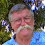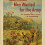## Saturday, April 2, 2016

### A LITTLE PI GOES A LONG WAY

Someone asked the Jet Propulsion Lab how much pi they used in their orbital calculations. Here's the answer provided by the  director and chief engineer for NASA's Dawn mission, Marc Rayman.
Here's what he said: To start, let me answer your question directly. For JPL's highest accuracy calculations, which are for interplanetary navigation, we use 3.141592653589793. Let's look at this a little more closely to understand why we don't use more decimal places. I think we can even see that there are no physically realistic calculations scientists ever perform for which it is necessary to include nearly as many decimal points as you present. Consider these examples:
The most distant spacecraft from Earth is Voyager 1. It is about 12.5 billion miles away. Let's say we have a circle with a radius of exactly that size (or 25 billion miles in diameter) and we want to calculate the circumference, which is pi times the radius times 2. Using pi rounded to the 15th decimal, as I gave above, that comes out to a little more than 78 billion miles. We don't need to be concerned here with exactly what the value is (you can multiply it out if you like) but rather what the error in the value is by not using more digits of pi. In other words, by cutting pi off at the 15th decimal point, we would calculate a circumference for that circle that is very slightly off. It turns out that our calculated circumference of the 25 billion mile diameter circle would be wrong by 1.5 inches. Think about that. We have a circle more than 78 billion miles around, and our calculation of that distance would be off by perhaps less than the length of your little finger.
We can bring this down to home with our planet Earth. It is 7,926 miles in diameter at the equator. The circumference then is 24,900 miles. That's how far you would travel if you circumnavigated the globe (and didn't worry about hills, valleys, obstacles like buildings, rest stops, waves on the ocean, etc.). How far off would your odometer be if you used the limited version of pi above? It would be off by the size of a molecule. There are many different kinds of molecules, of course, so they span a wide range of sizes, but I hope this gives you an idea. Another way to view this is that your error by not using more digits of pi would be 10,000 times thinner than a hair!
Let's go to the largest size there is: the visible universe. The radius of the universe is about 46 billion light years. Now let me ask a different question: How many digits of pi would we need to calculate the circumference of a circle with a radius of 46 billion light years to an accuracy equal to the diameter of a hydrogen atom (the simplest atom)? The answer is that you would need 39 or 40 decimal places. If you think about how fantastically vast the universe is — truly far beyond what we can conceive, and certainly far, far, far beyond what you can see with your eyes even on the darkest, most beautiful, star-filled night — and think about how incredibly tiny a single atom is, you can see that we would not need to use many digits of pi to cover the entire range.
So, there you go, a little pi goes a long way.

1.I like pi.

(Great article too.)

1.2.2.1.